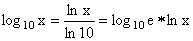About RF CafeCopyright: 1996 - 2024Webmaster:     BSEE - KB3UON RF Cafe began life in 1996 as "RF Tools" in an AOL screen name web space totaling 2 MB. Its primary purpose was to provide me with ready access to commonly needed formulas and reference material while performing my work as an RF system and circuit design engineer. The World Wide Web (Internet) was largely an unknown entity at the time and bandwidth was a scarce commodity. Dial-up modems blazed along at 14.4 kbps while tying up your telephone line, and a nice lady's voice announced "You've Got Mail" when a new message arrived... All trademarks, copyrights, patents, and other rights of ownership to images and text used on the RF Cafe website are hereby acknowledged. My Hobby Website: AirplanesAndRockets.com# Laws of Logarithms

According to the 1797 Britannica a logarithm is:

"...a series of numbers in arithmetical progression, corresponding to others in geometrical progression; by means of which, arithmetical calculations can be made with much more ease and expedition than otherwise."

This fundamental property of logarithms makes them utterly indispensible in engineering and science, where often it is necessary to "mentally" multiply an divide quantities like gain, noise figure, etc. Thanks to logarithms, those multiplication and division operations are transformed into much simpler tasks of addition and subtract, respectively.

To make the process even simpler, many of our engineering and science quantities are routinely expressed in units of decibels. We work with decibels (dB) of gain, decibels wrt a milliwatt of power (dBm), and many other such quantities. Decibels are commonly used in optics, acoustics, and other realms of physics.

 Basic Rules If x = ay, then y = loga xloga (x * y) = loga x + loga yloga (x / y) = loga x - loga yloga (xn) = n * loga x Change of Baseso,In General: log10 x is written as log x loge x is written as ln x      where "e" is the base of the natural logarithm

An example would be where you have three stages of gain in series (cascade) that need to be totaled. Supposed that the first stage quadrupled the power of the input signal (gain = 4), the second stage increased the power by a factor of 20 (gain = 20), the third stage increased the power by a factor of 2 (gain = 2). In order to calculate the total power gain, you multiply the gains for a total of 4 x 20 x 2  = 160.

Now, that is not such a hard mental exercise, but suppose instead the stage gains were as follows:

g1 = 7.51

g2 = 22.80

g3 = 3.94

Quick, what is the total gain? If you are a math whiz, you would immediately answer, "674.63832." If you were a disciplined scientist, however, you would reply, "675," because you would dutifully you know that the answer cannot be of any greater precision than the lowest precision of any involved quantity. But, I digress.

Now, let us go about the same exercise using decibels.

G1 = 10 log10 (7.51) = 8.76 dB

G2 = 10 log10 (22.80) = 13.58 dB

G3 = 10 log10 (3.94) = 5.95 dB

Total gain is the sum of the three gains = 28.29 dB (ok, 28.3 dB)

To check the results: 10 log10 (674.63832) = 28.3 dB   Q.E.D., as they say.Please Support RF Cafe by purchasing my  ridiculously low−priced products, all of which I created. These Are Available for Free